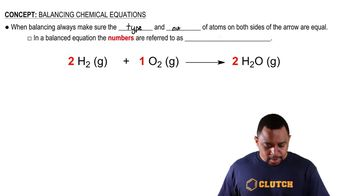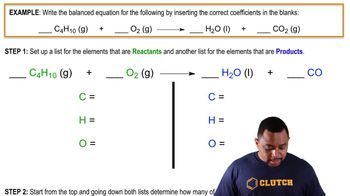Start typing, then use the up and down arrows to select an option from the list.
1:46 minutes
Problem 10
Textbook Question

# A key step in balancing chemical equations is correctly identifying the formulas of the reactants and products. For example, consider the reaction between calcium oxide, CaO(s), and H2O1l2 to form aqueous calcium hydroxide. (b) Is it possible to balance the equation if you incorrectly identify the product as CaOH1aq2, and if so, what is the equation?Verified Solution
This video solution was recommended by our tutors as helpful for the problem above.
224views